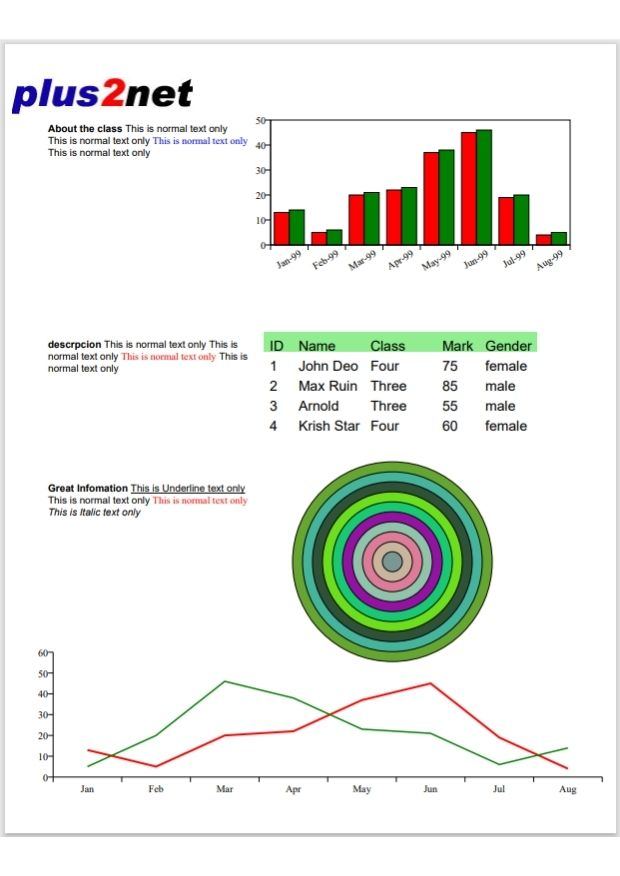# PDF report using image, charts and Paragraphs

PDF Shapes

Creating PDF report by using charts, table, graphs with text paragraphs by combination of components

We are adding five different components to our report. We created the images , charts, graphs and paragraphs separately and imported them to our main.py file. ( download the Zip file below with all source codes/ files )
``````from chart_verticalbar_chart import d
from table_1_basic import t
from chart_linechart import line_chart
from paragraph1 import p1,p2,p3
from chart_2_circle import d_circle``````
We set the path with file name in our main.py file and this file finally creates the PDF document.
``my_path='G:\\My drive\\testing\\pypdf2\\my_pdf.pdf'``Change the path of image here.
.
``c.drawImage('D:\\top2.jpg',.1*inch,10*inch)  # image at left side top``

## Adding Vertical Bar chart

``````from chart_verticalbar_chart import d
d.wrapOn(c,400,400)
d.drawOn(c, 3*inch,7.5*inch)   # vertical bar chart ``````

``````from table_1_basic import t
t.wrapOn(c, width, height)
t.drawOn(c,3.6*inch, 5.6*inch) # table at right side``````

``````from paragraph1 import p1,p2,p3
p1.wrapOn(c, 200, 200)
p1.drawOn(c, .6*inch,9.4*inch) # top paragraph of text

p2.wrapOn(c, 200, 200)
p2.drawOn(c, .6*inch,6.4*inch) # middle paragraph of text

p3.wrapOn(c, 200, 200)
p3.drawOn(c, .6*inch,4.4*inch) # last paragraph of text ``````

## Adding shapes ( Circles )

``````from chart_2_circle import d_circle
d_circle.wrapOn(c, 100, 100)
d_circle.drawOn(c, 4*inch,2.4*inch) # circles drawn at right ``````

## Adding Line chart

``````from chart_linechart import line_chart
line_chart.wrapOn(c, 810, 200)
line_chart.drawOn(c, .6*inch,.1*inch) # line chart at bottom``````
Full code is here.

main.py
``````from reportlab.pdfgen import canvas
from reportlab.lib.pagesizes import letter
from reportlab.lib.units import inch

from chart_verticalbar_chart import d
from table_1_basic import t
from chart_linechart import line_chart
from paragraph1 import p1,p2,p3
from chart_2_circle import d_circle

my_path='G:\\My drive\\testing\\pypdf2\\my_pdf.pdf'
c = canvas.Canvas(my_path, pagesize=letter)
width, height = letter

c.drawImage('D:\\top2.jpg',.1*inch,10*inch)  # image at left side top
d.wrapOn(c,400,400)
d.drawOn(c, 3*inch,7.5*inch)   # vertical bar chart

t.wrapOn(c, width, height)
t.drawOn(c,3.6*inch, 5.6*inch) # table at right side

p1.wrapOn(c, 200, 200)
p1.drawOn(c, .6*inch,9.4*inch) # top paragraph of text

p2.wrapOn(c, 200, 200)
p2.drawOn(c, .6*inch,6.4*inch) # middle paragraph of text

p3.wrapOn(c, 200, 200)
p3.drawOn(c, .6*inch,4.4*inch) # last paragraph of text

d_circle.wrapOn(c, 100, 100)
d_circle.drawOn(c, 4*inch,2.4*inch) # circles drawn at right

line_chart.wrapOn(c, 810, 200)
line_chart.drawOn(c, .6*inch,.1*inch) # line chart at bottom
c.save()``````

Subscribe to our YouTube Channel here

## Subscribe

* indicates required
Subscribe to plus2netplus2net.com

Post your comments , suggestion , error , requirements etc here